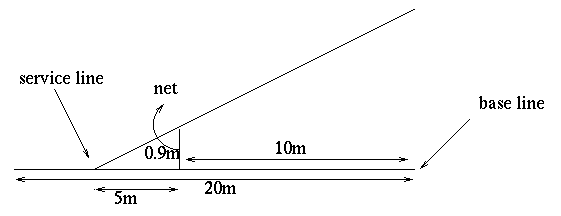#### You may also like### How Big?

If the sides of the triangle in the diagram are 3, 4 and 5, what is the area of the shaded square?### Hex

Explain how the thirteen pieces making up the regular hexagon shown in the diagram can be re-assembled to form three smaller regular hexagons congruent to each other.### Triangular Tantaliser

Draw all the possible distinct triangles on a 4 x 4 dotty grid. Convince me that you have all possible triangles.

# Tennis

##### Age 11 to 14 Challenge Level:

On a given tennis court, the distance between the baseline and the net is $10$ metres and the distance between the service line and the net is $5$ metres. The net is $0.9$ metres high. A player serves the ball directly above the baseline (assume the ball travels in a straight line).1. What is the minimum height that the ball can be hit at so as to ensure the ball lands in the service area?
2. How far does the ball travel before it hits the ground?
3. Assuming the player receiving the serve stays on the baseline and hits the ball as it crosses the baseline, how should the server hit the ball so that his opponent has the least time to receive the serve?
This problem was given by Mikael Brown.